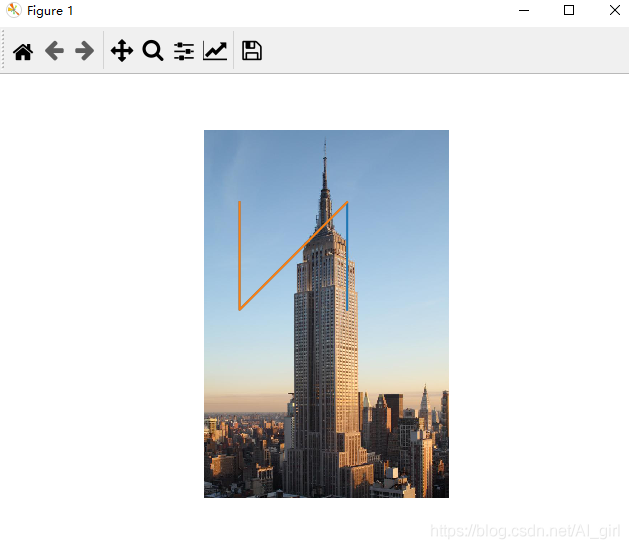# python圖像處理基本操作總結（PIL庫、Matplotlib及Numpy）

### 一、PIL庫對圖像的基本操作

1、讀取圖片

PIL網上有很多介紹，這裡不再講解。直接操作，讀取一張圖片，將其轉換為灰度圖像，並打印出來。

```from  PIL  import Image
import matplotlib.pyplot as plt
pil_im = Image.open("empire.jpeg")
pil_image = pil_im.convert("L")
plt.gray()
plt.imshow(pil_image)
plt.show()```2、轉換圖片格式

PIL可以將圖像保存為多種格式，下面將PNG格式文件保存為JPG格式：

```from PIL import Image
import glob
import os
filelist = glob.glob("E:/pythonProject1/filelist/*.png")
for infile in filelist:
outfile = os.path.splitext(infile)+'.jpg'

if infile  != outfile:
try:
Image.open(infile).save(outfile)
except IOError:
print("cannot convert", infile)```3、輸出文件夾中所有圖片的文件名列表

```import os
def get_imlist(path):
"""返回目錄中所有JPG圖像的文件名列表"""
return [os.path.join(path,f)for f in os.listdir(path) if f.endswith('.jpg')]
print(get_imlist("E:/pythonProject1/filelist/"))```

### 二、Matplotlib

1、繪制圖像、點和線

```from PIL import Image
from pylab import *

#讀取圖像到數組中
im = array(Image.open("empire.jpeg"))

#繪制圖像
imshow(im)

#一些點
x = [100, 100, 400, 400]
y = [200, 500, 200, 500]

#使用紅色星狀標記繪制點
plot(x, y)#默認為藍色實線
# plot(x, y, 'r*')#紅色星狀標記
# plot(x, y, 'go-')#帶有圓圈標記的綠線
# plot(x, y, 'ks')#帶有正方形標記的黑色虛線

#繪制連接前三個點的線
plot(x[:3], y[:3])
axis('off')

#添加標題，顯示繪制的圖像
titles = ['empire']
plt.title = titles
show()```2、圖像輪廓和直方圖

```from PIL import Image
from pylab import *

# 讀取圖像到數組中
im = array(Image.open("empire.jpeg").convert('L'))

#創建一個圖像
figure()
#不使用顏色信息
gray()
#在原點的左上角顯示輪廓圖像
contour(im, origin = 'image')#檢測圖像輪廓
axis('equal')
axis('off')
show()
#新建一個圖像
figure
hist(im.flatten(), 128)#繪制圖像直方圖
show()```3、交互式標註

```from PIL import Image
from pylab import *

im = array(Image.open("empire.jpeg"))
imshow(im)
print("please click 3 points")
x = ginput(3)
print("you clicked",x)
show()```

### 三、Numpy

1、圖像數組表示

```from PIL import Image
import numpy as np

im = np.array(Image.open("empire.jpeg"))
print(im.shape,im.dtype)```

(1024, 683, 3) uint8

每行的第一個元組表示圖像數組的大小（行、列、顏色通道），緊接著的字符串表示數組元素的數據類型。因為圖像通常被編碼成無符號八位整數（uint8），載入圖像並將其轉換到數組中，數組的數據類型為“uint8”。

2、灰度變換

```from PIL import Image
import numpy as np

im = np.array(Image.open("empire.jpeg"))
print(im.shape,im.dtype)

from PIL import Image
from matplotlib.pylab import plt
from numpy import *

im1 = array(Image.open('empire.jpeg').convert('L'))
im2 = 255 - im1 #對圖像進行反向處理
im3 = (100.0/255) * im1 + 100 #將圖像值變換到100-200之間
im4 = 255.0 * (im1/255) ** 2 #對圖像像素值求平方後得到的圖像

images = [im1, im2, im3, im4]
titles = ["f(x) = x", "f(x) = 255 - x", "f(x) = (100/255)*x +100", "f(x) = 255*(x/255)^2"]
#輸出圖中的最大像素值和最小像素值
print(int(im1.min()),int(im1.max()))
print(int(im2.min()),int(im2.max()))
print(int(im3.min()),int(im3.max()))
print(int(im4.min()),int(im4.max()))

for i in range(4):
plt.subplot(2, 2, i+1)#2行2列，按編號順序排列
plt.imshow(images[i])#顯示圖像
plt.title(titles[i])#顯示標題
plt.gray()
# plt.xticks([])
# plt.yticks([])
plt.axis('equal')
plt.axis('off')
plt.show()```